p-block – JEE Advanced Previous Year Questions with Solutions
JEE Advanced Previous Year Questions of Chemistry with Solutions are available at eSaral. Practicing JEE Advanced Previous Year Papers Questions of Chemistry will help the JEE aspirants in realizing the question pattern as well as help in analyzing weak & strong areas.Simulator Previous Years JEE Advance Questions
Q. White phosphorus on reaction with NaOH gives $\mathrm{PH}_{3}$ as one of the products. This is a :-(A) dimerization reaction(B) disproportionation reaction(C) condensation reaction(D) precipitation reaction [JEE 2009]

Download eSaral App for Video Lectures, Complete Revision, Study Material and much more...

Sol. (B)$\mathrm{P}_{4}+\mathrm{NaOH} \rightarrow \mathrm{PH}_{3}+\mathrm{NaH}_{2} \mathrm{PO}_{2}$(Disproportionation reaction)

Q. The reaction of $P_{4}$ with X leads selectively to $\mathrm{P}_{4} \mathrm{O}_{6}$. The X is(A) Dry $\mathrm{O}_{2}$(B) A mixture of $\mathrm{O}_{2},$ and $\mathrm{N}_{2}$(C) Moist $\mathrm{O}_{2}$(D) $\mathrm{O}_{2}$ in the presence of aqueous $\mathrm{NaOH}$ [JEE 2009]

Download eSaral App for Video Lectures, Complete Revision, Study Material and much more...

Sol. (B)$\mathrm{P}_{4}+\left(\mathrm{O}_{2}+\mathrm{N}_{2}\right) \stackrel{\Delta}{\longrightarrow} \mathrm{P}_{4} \mathrm{O}_{6}+\mathrm{N}_{2}$

Q. The nitrogen oxide(s) that contain(s) N–N bond(s) is (are)(A) $\mathrm{N}_{2} \mathrm{O}$(B) $\mathrm{N}_{2} \mathrm{O}_{3}$ (unsym)(C) $\mathrm{N}_{2} \mathrm{O}_{4}$(D) $\mathrm{N}_{2} \mathrm{O}_{5}$ [JEE 2009]

Download eSaral App for Video Lectures, Complete Revision, Study Material and much more...

Sol. (A,B,C)Q. In the reaction, $2 \mathrm{X}+\mathrm{B}_{2} \mathrm{H}_{6} \longrightarrow\left[\mathrm{BH}_{2}(\mathrm{X})_{2}\right]^{+}\left[\mathrm{BH}_{4}\right]^{-}$ the amine(s) X is (are)(A) $\mathrm{NH}_{3}$(B) $\mathrm{CH}_{3} \mathrm{NH}_{2}$(C) $\left(\mathrm{CH}_{3}\right)_{2} \mathrm{NH}$(D) $\left(\mathrm{CH}_{3}\right)_{3} \mathrm{N}$ [JEE 2009]

Download eSaral App for Video Lectures, Complete Revision, Study Material and much more...

Sol. (B,C)$2 \mathrm{X}+\mathrm{B}_{2} \mathrm{H}_{6} \longrightarrow\left[\mathrm{BH}_{2}(\mathrm{X})_{2}\right]^{+}\left[\mathrm{BH}_{4}\right]^{-}$

Q. The reaction of white phosphorus with aqueous NaOH gives phosphine along with another phosphorus containing compound. The reaction type ; the oxidation states of phosphorus in phosphine and the other product are respectively(A) redox reaction ; -3 and –5(B) redox reaction ; +3 and +5(C) disproportionation reaction ; -3 and +1(D) disproportionation reaction ; -3 and +3 [JEE 2012]

Download eSaral App for Video Lectures, Complete Revision, Study Material and much more...

Sol. (C)$\mathrm{P}_{4}+\mathrm{NaOH} \rightarrow^{-3} \mathrm{PH}_{3}+\mathrm{NaH}_{2} \mathrm{PO}_{2}$

Q. Bleaching powder contains a salt of an oxoacid as one of its components. The anhydride of that oxoacid is :(A) $\mathrm{Cl}_{2} \mathrm{O}$(B) $\mathrm{Cl}_{2} \mathrm{O}_{7}$(C) $\mathrm{ClO}_{2}$(D) $\mathrm{Cl}_{2} \mathrm{O}_{6}$ [JEE 2012]

Download eSaral App for Video Lectures, Complete Revision, Study Material and much more...

Sol. (A)Bleaching powder : $\mathrm{Ca}(\mathrm{OC} \ell) \mathrm{C} \ell$

Q. With respect to graphite and diamond, which of the statement(s) given below is (are) correct ?(A) Graphite is harder than diamond. (B) Graphite has higher electrical conductivity than diamond.(C) Graphite has higher thermal conductivity than diamond.(D) Graphite has higher C–C bond order than diamond. [JEE 2012]

Download eSaral App for Video Lectures, Complete Revision, Study Material and much more...

Sol. (B,D)

Q. Concentrated nitric acid, upon long standing, turns yellow-brown due to the formation of –(A) NO(B) $\mathrm{NO}_{2}$(C) $\mathrm{N}_{2} \mathrm{O}$(D) $\mathrm{N}_{2} \mathrm{O}_{4}$ [JEE 2013]

Download eSaral App for Video Lectures, Complete Revision, Study Material and much more...

Sol. (B)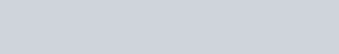Q. The correct statement(s) about $\mathrm{O}_{3}$ is(are)(A) O–O bond lengths are equal(B) Thermal decomposition of $\mathrm{O}_{3}$ is endothermic(C) $\mathrm{O}_{3}$ is diamagnetic in nature(D) $\mathrm{O}_{3}$ has a bent structure [JEE 2013]

Download eSaral App for Video Lectures, Complete Revision, Study Material and much more...

Sol. (A,C,D)Comprehension (Q. 10 and 11)The reaction of $\mathrm{Cl}_{2}$ gas with cold dilute and hot concentrated NaOH in water give sodium salt of two (different) oxoacids of chlorine P and Q respectively. The $\mathrm{Cl}_{2}$ gas reacts with $\mathrm$\mathrm{SO}_{2}$gas , in presence of charcoal to give a product R. R reacts with white phosphorous to give a compound S. On hydrolysis, S gives as oxoacid of phosphorous T. Q. R, S and T , respectively are –(A)$\mathrm{SO}_{2} \mathrm{Cl}_{2}, \mathrm{PCl}_{5}$and$\mathrm{H}_{3} \mathrm{PO}_{4}$(B)$\mathrm{SO}_{2} \mathrm{Cl}_{2}, \mathrm{PCl}_{3}$and$\mathrm{H}_{3} \mathrm{PO}_{3}$(C)$\mathrm{SOCl}_{2}, \mathrm{PCl}_{3}$and$\mathrm{H}_{3} \mathrm{PO}_{2}$(D)$\mathrm{SO}_{2} \mathrm{Cl}_{2}, \mathrm{PCl}_{5}$and$\mathrm{H}_{3} \mathrm{PO}_{4}$[JEE 2013] Download eSaral App for Video Lectures, Complete Revision, Study Material and much more... Sol. (A)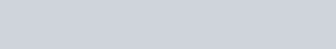Q. P and Q, respectively, are the sodium salts of – (A) Hypochlorus and chloric acid(B) Hypochlorus and chlorus acid(C) Chloric and perchloric acids(D) Chloric and hypochlorus acids [JEE 2013] Download eSaral App for Video Lectures, Complete Revision, Study Material and much more... Sol. (A) Q. The unbalanced chemical reactions given in List-I show missing reagent or condition (?) which are provided in List-II. Match List-I with List-II and select the correct answer using the code given below the lists :A[JEE 2013] Download eSaral App for Video Lectures, Complete Revision, Study Material and much more... Sol. (D)Q. Under ambient conditions, the total number of gases released as products in the final step of the reaction scheme shown below is(A) 0 (B) 1 (C) 2 (D) 3 [JEE Adv. 2014] Download eSaral App for Video Lectures, Complete Revision, Study Material and much more... Sol. (C)Q. The product formed in the reaction of$\mathrm{SOCl}_{2}$with white phosphorous is(A)$\mathrm{PCl}_{3}$(B)$\mathrm{SO}_{2} \mathrm{Cl}_{2}$(C)$\mathrm{SCl}_{2}$(D)$\mathrm{POCl}_{3}$[JEE Adv. 2014] Download eSaral App for Video Lectures, Complete Revision, Study Material and much more... Sol. (A)$\mathrm{P}_{4}+\mathrm{SOC} \ell_{2} \rightarrow \mathrm{PCl}_{3}+\mathrm{SO}_{2}+\mathrm{S}_{2} \mathrm{Cl}_{2}$Q. The correct statements(s) for orthoboric acid is / are –(A) It behaves as a weak acid in water due to self ionization(B) Acidity of its aqueous solution increases upon addition of ethylene glycol(C) It has a three dimensional structure due to hydrogen bonding.(D) It is a weak electrolyte in water [JEE Adv. 2014] Download eSaral App for Video Lectures, Complete Revision, Study Material and much more... Sol. (B,D)Q. The correct statement(s) regarding, (i) HClO, (ii)$\mathrm{HClO}_{2},$(iii)$\mathrm{HClO}_{3}$and (iv)$\mathrm{HClO}_{4},$is (are)(A) The number of Cl=O bonds in (ii) and (iii) together is two(B) The number of lone pairs of electrons on Cl in (ii) and (iii) together is three(C) The hybridization of$\mathrm{Cl}$in (iv) is sp$^{3}$(D) Amongst (i) to (iv), the strongest acid is (i) [JEE Adv. 2015] Download eSaral App for Video Lectures, Complete Revision, Study Material and much more... Sol. (B,C)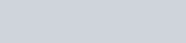Q. When$\mathrm{O}_{2}$is adsorbed on a metallic surface, electron transfer occurs from the metal to$\mathrm{O}_{2}$. The TRUE, statement (s) regarding this adsorption is (are)(A)$\mathrm{O}_{2}$is physisorbed(B) heat is released(C) occupancy of$\pi_{2 \mathrm{p}}^{*}$of$\mathrm{O}_{2}$is increased(D) bond length of$\mathrm{O}_{2}$is increased [JEE Adv. 2015] Download eSaral App for Video Lectures, Complete Revision, Study Material and much more... Sol. (B,C,D) Q. Under hydrolytic conditions, the compounds used for preparation of linear polymer and for chain termination, respectively, are(A)$\mathrm{CH}_{3} \mathrm{SiCl}_{3}$and$\mathrm{Si}\left(\mathrm{CH}_{3}\right)_{4}$(B)$\left(\mathrm{CH}_{3}\right)_{2} \mathrm{SiCl}_{2}$and$\left(\mathrm{CH}_{3}\right)_{3} \mathrm{SiCl}$(C)$\left(\mathrm{CH}_{3}\right)_{2} \mathrm{SiCl}_{2}$and$\mathrm{CH}_{3} \mathrm{SiCl}_{3}$(D)$\mathrm{SiCl}_{4}$and$\left(\mathrm{CH}_{3}\right)_{3} \mathrm{SiCl}$[JEE (Adv.) 2015] Download eSaral App for Video Lectures, Complete Revision, Study Material and much more... Sol. (B) Q. Three moles of$\mathrm{B}_{2} \mathrm{H}_{6}$are completely reacted with methanol. The number of moles of boron containing product formed is – [JEE (Adv.) 2015] Download eSaral App for Video Lectures, Complete Revision, Study Material and much more... Sol. 6 Q. The total number of lone pairs of electrons in$\mathrm{N}_{2} \mathrm{O}_{3}$is : [JEE Adv. 2015] Download eSaral App for Video Lectures, Complete Revision, Study Material and much more... Sol. 8 Q. The nitrogen containing compound produced in the reaction of$\mathrm{HNO}_{3}$with$\mathrm{P}_{4} \mathrm{O}_{10}$(A) can also be prepared by reaction of$\mathrm{P}_{4}$and$\mathrm{HNO}_{3}$(B) is diamagnetic(C) contains one N-N bond(D) reacts with Na metal producing a brown gas [JEE – Adv. 2016] Download eSaral App for Video Lectures, Complete Revision, Study Material and much more... Sol. (B,D)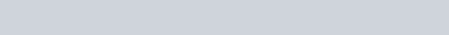Q. The increasing order of atomic radii of the following group 13 elements is(A) Al < Ga < In < Tl(B) Ga < Al < In < Tl(C) Al < In < Ga < Tl(D) Al < Ga < Tl < In [JEE Adv. 2016] Download eSaral App for Video Lectures, Complete Revision, Study Material and much more... Sol. (B)The order of radius of 13th group elements is Ga < Al < In < Tl.Reason Due to poor shielding effect of d-orbital, radius of Ga is smallar than Al. Q. The crystalline form of borax has(A) Tetranuclear$\left[\mathrm{B}_{4} \mathrm{O}_{5}(\mathrm{OH})_{4}\right]^{2-}$unit(B) All boron atoms in the same plane(C) Equal number of$\mathrm{sp}^{2}$and$\mathrm{sp}^{3}$hybridized boron atoms(D) One terminal hydroxide per boron atom [JEE Adv. 2016] Download eSaral App for Video Lectures, Complete Revision, Study Material and much more... Sol. (A,C,D)(A) Having$\left[\mathrm{B}_{4} \mathrm{O}_{5}(\mathrm{OH})_{4}\right]^{2-}$tetranuclear (boron) unit(B) All boron atoms not in same plane(C) Two boron are$\mathrm{sp}^{2}$hybridised and two boron are$\mathrm{sp}^{3}$hybridised(D) One terminal hydroxide per boron atom is present. Paragraph for Q.24 & 25Upon heating$\mathrm{KClO}_{3}$in the presence of catalytic amount of$\mathrm{MnO}_{2}$, a gas W is formed. Excess amount of W reacts with whitephosphorus to give X. The reaction of X with pure$\mathrm{HNO}_{3}$gives Y and Z. Q. W and X are, respectively(A)$\mathrm{O}_{3}$and$\mathrm{P}_{4} \mathrm{O}_{6}$(B)$\mathrm{O}_{2}$and$\mathrm{P}_{4} \mathrm{O}_{10}$(C)$\mathrm{O}_{3}$and$\mathrm{P}_{4} \mathrm{O}_{10}$(D)$\mathrm{O}_{2}$and$\mathrm{P}_{4} \mathrm{O}_{6}$[JEE – Adv. 2017] Download eSaral App for Video Lectures, Complete Revision, Study Material and much more... Sol. (B) Q. Y and Z are , respectively(A)$\mathrm{N}_{2} \mathrm{O}_{4}$and$\mathrm{H}_{3} \mathrm{PO}_{3}$(B)$\mathrm{N}_{2} \mathrm{O}_{4}$and$\mathrm{HPO}_{3}$(C)$\mathrm{N}_{2} \mathrm{O}_{5}$and$\mathrm{HPO}_{3}$(D)$\mathrm{N}_{2} \mathrm{O}_{3}$and$\mathrm{H}_{3} \mathrm{PO}_{4}$[JEE – Adv. 2017] Download eSaral App for Video Lectures, Complete Revision, Study Material and much more... Sol. (C) Q. The correct statements(s) about the oxoacids,$\mathrm{HClO}_{4}$and$\mathrm{HClO},$is (are) –(A)$\mathrm{HClO}_{4}$is more acidic than HClO because of the resonance stabilization of its anion(B)$\mathrm{HClO}_{4}$is formed in the reaction between$\mathrm{Cl}_{2}$and$\mathrm{H}_{2} \mathrm{O}$(C) The central atom in Both$\mathrm{HClO}_{4}$and HClO is$\mathrm{sp}^{3}$hybridized(D) The conjugate base of$\mathrm{HClO}_{4}$is weaker base than$\mathrm{H}_{2} \mathrm{O}$[JEE – Adv. 2017] Download eSaral App for Video Lectures, Complete Revision, Study Material and much more... Sol. (A,C,D)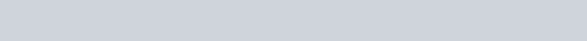Q. The colour of the$\mathrm{X}_{2}$molecules of group 17 elements changes gradually from yellow to violet down the group. This is due to –(A) the physical state of$\mathrm{X}_{2}$at room temperature changes from gas to solid down the group(B) decrease in HOMO-LUMO gap down the group(C) decrease in$\pi^{*}-\sigma^{*}$down the group(D) decrease in ionization energy down the group [JEE – Adv. 2017] Download eSaral App for Video Lectures, Complete Revision, Study Material and much more... Sol. (B,C)Halogens are coloured due to HOMO-LUMO transition of electrons.On moving down the group HOMO-LUMO energy gap decreases so transition of electrons become easier$\stackrel{*}{\pi} 2 \mathrm{p}$to$\stackrel{*}{\sigma} 2 \mathrm{p}$therefore colour intensify. Q. The order of the oxidation state of the phosphorus atom in$\mathrm{H}_{3} \mathrm{PO}_{2}, \mathrm{H}_{3} \mathrm{PO}_{4}, \mathrm{H}_{3} \mathrm{PO}_{3}$and$\mathrm{H}_{4} \mathrm{P}_{2} \mathrm{O}_{6}$is (A)$\mathrm{H}_{3} \mathrm{PO}_{4}>\mathrm{H}_{4} \mathrm{P}_{2} \mathrm{O}_{6}>\mathrm{H}_{3} \mathrm{PO}_{3}>\mathrm{H}_{3} \mathrm{PO}_{2}$(B)$\mathrm{H}_{3} \mathrm{PO}_{3}>\mathrm{H}_{3} \mathrm{PO}_{2}>\mathrm{H}_{3} \mathrm{PO}_{4}>\mathrm{H}_{4} \mathrm{P}_{2} \mathrm{O}_{6}$(C)$\mathrm{H}_{3} \mathrm{PO}_{2}>\mathrm{H}_{3} \mathrm{PO}_{3}>\mathrm{H}_{4} \mathrm{P}_{2} \mathrm{O}_{6}>\mathrm{H}_{3} \mathrm{PO}_{4}$(D)$\mathrm{H}_{3} \mathrm{PO}_{4}>\mathrm{H}_{3} \mathrm{PO}_{2}>\mathrm{H}_{3} \mathrm{PO}_{3}>\mathrm{H}_{4} \mathrm{P}_{2} \mathrm{O}_{6}$[JEE – Adv. 2017] Download eSaral App for Video Lectures, Complete Revision, Study Material and much more... Sol. (A)Q. The option(s) with only amphoteric oxides is (are):(A)$\mathrm{Cr}_{2} \mathrm{O}_{3}, \mathrm{CrO}, \mathrm{SnO}, \mathrm{PbO}$(B)$\mathrm{NO}, \mathrm{B}_{2} \mathrm{O}_{3}, \mathrm{PbO}, \mathrm{SnO}_{2}$(C)$\mathrm{Cr}_{2} \mathrm{O}_{3}, \mathrm{BeO}, \mathrm{SnO}, \mathrm{SnO}_{2}$(D)$\mathrm{ZnO}, \mathrm{Al}_{2} \mathrm{O}_{3}, \mathrm{PbO}, \mathrm{PbO}_{2}$[JEE – Adv. 2017] Download eSaral App for Video Lectures, Complete Revision, Study Material and much more... Sol. (C,D)Q. Among the following, the correct statement(s) is are(A)$\mathrm{Al}\left(\mathrm{CH}_{3}\right)_{3}$has the three-centre two-electron bonds in its dimeric structure(B)$\mathrm{AlCl}_{3}$has the three-centre two-electron bonds in its dimeric structure(C)$\mathrm{BH}_{3}$has the three-centre two-electron bonds in its dimeric structure(D) The Lewis acidity of$\mathrm{BCl}_{3}$is greater than that of$\mathrm{AlCl}_{3}$[JEE – Adv. 2017] Download eSaral App for Video Lectures, Complete Revision, Study Material and much more... Sol. (A,C,D)(A)(B)(C)(D) (D) Lewis acidic strength decreases down the group. The decrease in acid strength occurs because as size increases, the attraction between the incoming electron pair and the nucleus weakens. Hence Lewis acidic strength of$\mathrm{BCl}_{3}$is more than$\mathrm{AlCl}_{3}$. Q. The compound(s) which generate(s)$\mathrm{N}_{2}$gas upon thermal decomposition below$300^{\circ} \mathrm{C}$is (are)(A)$\mathrm{NH}_{4} \mathrm{NO}_{3}$(B)$\left(\mathrm{NH}_{4}\right)_{2} \mathrm{Cr}_{2} \mathrm{O}_{7}$(C)$\mathrm{Ba}\left(\mathrm{N}_{3}\right)_{2}(\mathrm{D}) \mathrm{Mg}_{3} \mathrm{N}_{2}$[JEE – Adv. 2018] Download eSaral App for Video Lectures, Complete Revision, Study Material and much more... Sol. (B,C)Q. Based on the compounds of group 15 elements , the correct statement(s) is (are)(A)$\mathrm{Bi}_{2} \mathrm{O}_{5}$is more basic than$\mathrm{N}_{2} \mathrm{O}_{5}$(B)$\mathrm{NF}_{3}$is more covalent than$\mathrm{BiF}_{3}$(C)$\mathrm{PH}_{3}$boils at lower temperature than$\mathrm{NH}_{3}$(D) The$\mathrm{N}-\mathrm{N}$single bond is stronger than the$\mathrm{P}-\mathrm{P}$single bond [JEE – Adv. 2018] Download eSaral App for Video Lectures, Complete Revision, Study Material and much more... Sol. (A,B,C)(A)$\mathrm{Bi}_{2} \mathrm{O}_{5}$is metallic oxide but$\mathrm{N}_{2} \mathrm{O}_{5}$is non metallic oxide therefore$\mathrm{Bi}_{2} \mathrm{O}_{5}$is basic but$\mathrm{N}_{2} \mathrm{O}_{5}$is acidic.(B) In$\mathrm{NF}_{3}$, N and F are non metals but$\mathrm{BiF}_{3}$, Bi is metal but F is non metal therefore$\mathrm{NF}_{3}$is more covalent than$\mathrm{BiF}_{3}$.(C) In$\mathrm{PH}_{3}$hydrogen bonding is absent but in$\mathrm{NH}_{3}$hydrogen bonding is present therefore$\mathrm{PH}_{3}$boils at lower temperature than$\mathrm{NH}_{3}$.(D) Due to small size in N–N single bond l.p. – l.p. repulsion is more than P–P single bond therefore N–N single bond is weaker than the P–P single bond. Q. The total number of compounds having at least one bridging oxo group among the molecules given below is______.$\mathrm{N}_{2} \mathrm{O}_{3}, \mathrm{N}_{2} \mathrm{O}_{5}, \mathrm{P}_{4} \mathrm{O}_{6}, \mathrm{P}_{4} \mathrm{O}_{7}, \mathrm{H}_{4} \mathrm{P}_{2} \mathrm{O}_{5}, \mathrm{H}_{5} \mathrm{P}_{3} \mathrm{O}_{10}, \mathrm{H}_{2} \mathrm{S}_{2} \mathrm{O}_{3}, \mathrm{H}_{2} \mathrm{S}_{2} \mathrm{O}_{5}\$ [JEE – Adv. 2018]

Download eSaral App for Video Lectures, Complete Revision, Study Material and much more...

Sol. 5 or 6